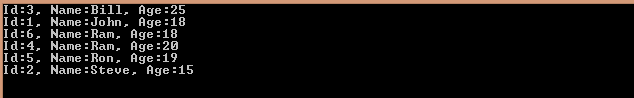# Use ThenBy() in Linq Query.```using System;using System.Collections.Generic;using System.Linq;using System.Text;namespace Prototype{    class Example    {        static void Main()        {            IList<employee> List = new List<employee>() {             new employee() { id = 1, empname = 'John', age = 18 } ,            new employee() { id = 2, empname = 'Steve',  age = 15 } ,            new employee() { id = 3, empname = 'Bill',  age = 25 } ,            new employee() { id = 4, empname = 'Ram' , age = 20 } ,            new employee() { id = 5, empname = 'Ron' , age = 19 },             new employee() { id = 6, empname = 'Ram' , age = 18 }               };